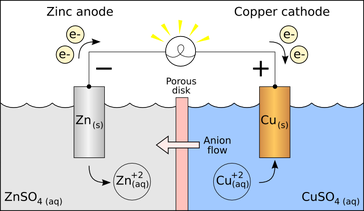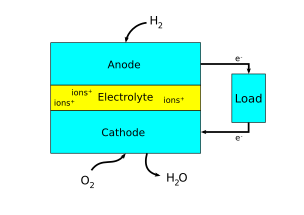ElectrodeFigure 1. A simplified diagram of a voltaic cell with zinc and copper electrodes to complete the circuit through a nonmetallic medium.

An electrode is a conductor that is used to make contact with a nonmetallic part of a circuit. Electrodes are commonly used in electrochemical cells (see Figure 1), semiconductors like diodes, and in medical devices. The electrode is the place where electron transfer occurs.

An electrode is classified as either a cathode or an anode depending on the type of chemical reaction that occurs. If an oxidation reaction occurs at an electrode (oxidation being the loss of electrons), then the electrode is classified as an anode. If a reduction reaction occurs at an electrode (reduction being the gain of electrons), then the electrode is classified as a cathode. Conventional current, in something like a discharging battery, flows into a device through its anode and leaves the device through the cathode.

The distinction can be made between active electrodes and inert electrodes. For example, a magnesium electrode is usually an active electrode because it participates in the oxidation-reduction (abbreviated as "redox") reaction. A platinum electrode is usually an inert electrode because it does not participate in the oxidation-reduction reaction. An inert electrode is chemically unreactive and is only present so that current can flow through the electrochemical cell.

Anode and CathodeFigure 2. A simplified diagram showing the anode and cathode of a fuel cell. Note that the arrows in the diagram show electron flow. Conventional current would be in the opposite direction.

There are many ways to think about which electrode is the anode and which is the cathode in an electrochemical system. Sometimes, the anodes and cathodes are described as negative and positive electrodes. However, this can be confusing since anodes and cathodes can both be negative or positive depending on whether the electrochemical cell is producing electricity or consuming electricity. Thus, the most helpful way to think about it is in relation to electron flow. As said earlier, the anode refers to an electrode where oxidation is taking place, or where electrons flow out. The cathode refers to an electrode where reduction is taking place, or where electrons flow in.

Anodes and cathodes are found in electrical components with a cell potential, including batteries, fuel cells, photovoltaic cells, electrolytic cells, and diodes.